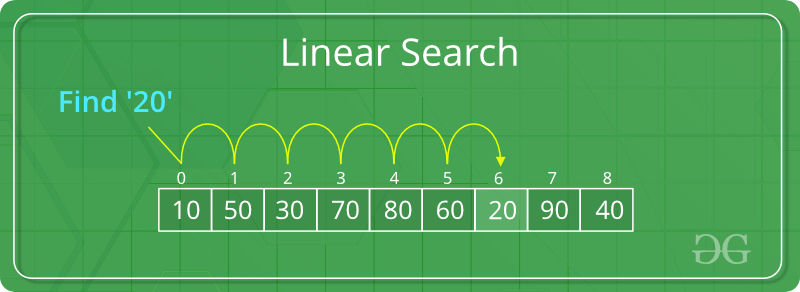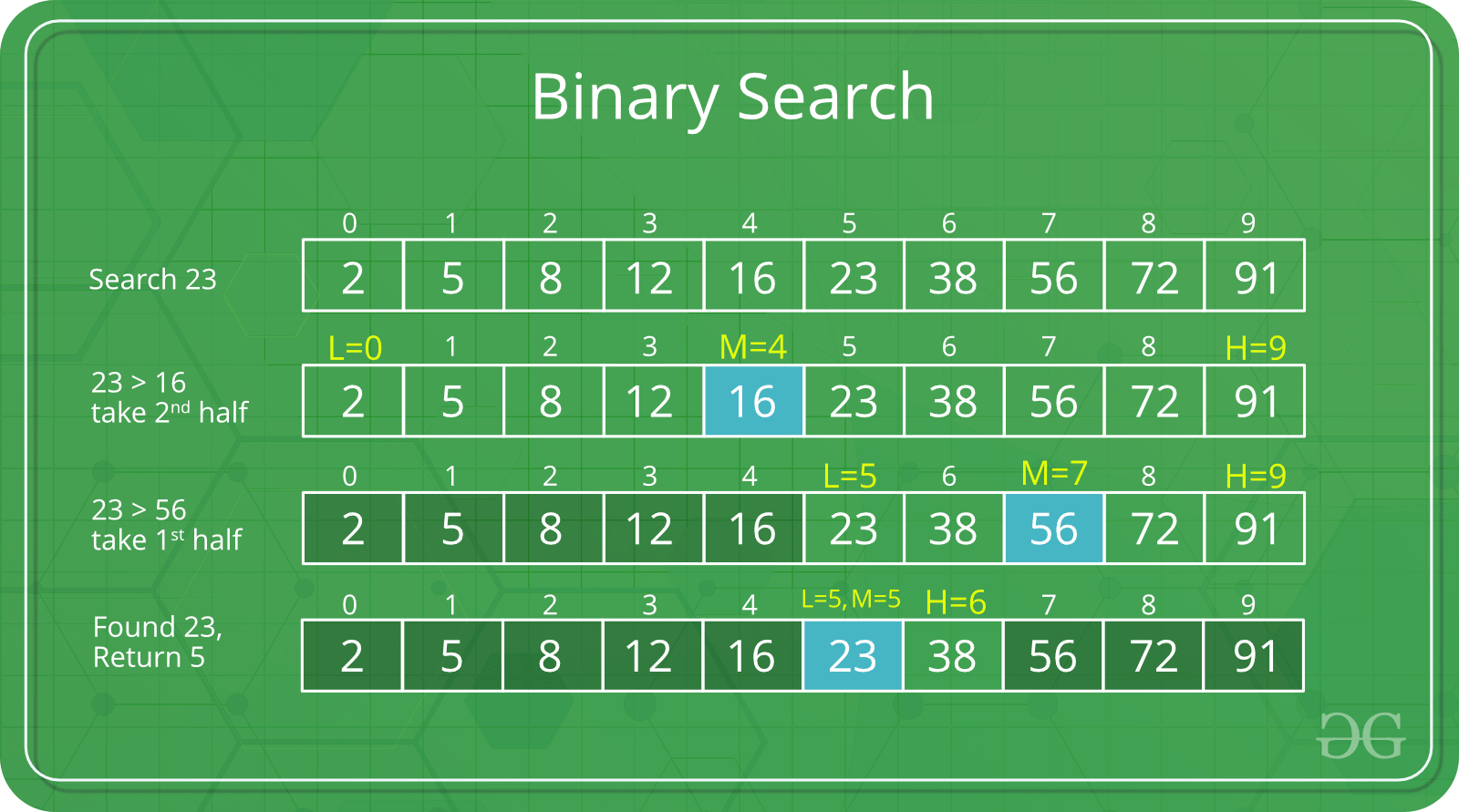Related Articles

# Difference between Searching and Sorting Algorithms

• Difficulty Level : Basic
• Last Updated : 20 Jul, 2021

Prerequisite: Searching and Sorting Algorithms

Searching Algorithms are designed to check for an element or retrieve an element from any data structure where it is used. Based on the type of operations these algorithms are generally classified into two categories:

1. Sequential Search: The Sequential Search is the basic and simple Searching Algorithm. Sequential Search starts at the beginning of the list or array. It traversed the list or array sequentially and checks for every element of the list or array. The Linear Search is an example of the Sequential Search.
A Linear Search checks one by one each element of the array, without jumping to any item. It searches the element in the array until a match is found. If the match is found then it returns the index of the item otherwise it returns the -1. The worst-case complexity of the Linear Search Algorithm is O(N), where N is the total number of elements in the list.

How Linear Search works:

Let’s understand with an example of how linear search works. Suppose, in this example, the task is to search an element x in the array. For searching the given element, start from the leftmost element of the array and one by one compare x with each element of the array. If the x matches with the an element it returns the index otherwise it returns the -1. Below is the image to illustrate the same:1. Interval Search: These algorithms are designed to searching for a given element in sorted data structures. These types of searching algorithms are much more efficient than a Linear Search Algorithm. The Binary Search is an example of the Interval Search.
A Binary Search searches the given element in the array by dividing the array into two halves. First, it finds the middle element of the array and then compares the given element with the middle element of thearray, if the element to be searched is less than the item in the middle of the array then the given element can only lie in the left subarray otherwise it lies in the right subarray. It repeatedly checks until the element is found. The worst-case complexity of the Binary Search Algorithm is O(log N)

How Binary Search works:
Let’s understand with an example of how Binary search works. Suppose, in this example we have to search a element x in the array. For searching the given element, first we find the middle element of the array then compare x with the middle element of the array. If x matches with the middle element we return the mid index and if x is greater then the mid element then x can be only lie in the right half subarray after the mid element, so we recur for the right half otherwise recur for thr left half. It repeatedly checks until the element is found. Below is the image to illustrate the same:Sorting Algorithm: A Sorting Algorithm is used to arranging the data of list or array into some specific order. It can be numerical or lexicographically order. For Example: The below list of characters is sorted in increasing order of their ASCII values. That is, the character with lesser ASCII value will be placed first than the character with higher ASCII value. The Bubble Sort, Insertion Sort, Selection Sort, Merge Sort, Quick Sort, Heap Sort, Radix Sort, etc are the examples of Sorting Algorithms.

There are two different categories in sorting. They are:

• Internal Sorting: When all data is placed in memory, then sorting is called internal sorting.
• External Sorting: When all data that needs to be sorted cannot be placed in memory at a time, the sorting is called External Sorting. External Sorting is used for massive amount of data. Merge Sort and its variations are typically used for external sorting. Some external storage like hard-disk, CD, etc is used for external storage.

### Difference between Searching and Sorting Algorithm:

Attention reader! Don’t stop learning now. Get hold of all the important DSA concepts with the DSA Self Paced Course at a student-friendly price and become industry ready.  To complete your preparation from learning a language to DS Algo and many more,  please refer Complete Interview Preparation Course.

In case you wish to attend live classes with experts, please refer DSA Live Classes for Working Professionals and Competitive Programming Live for Students.

My Personal Notes arrow_drop_up